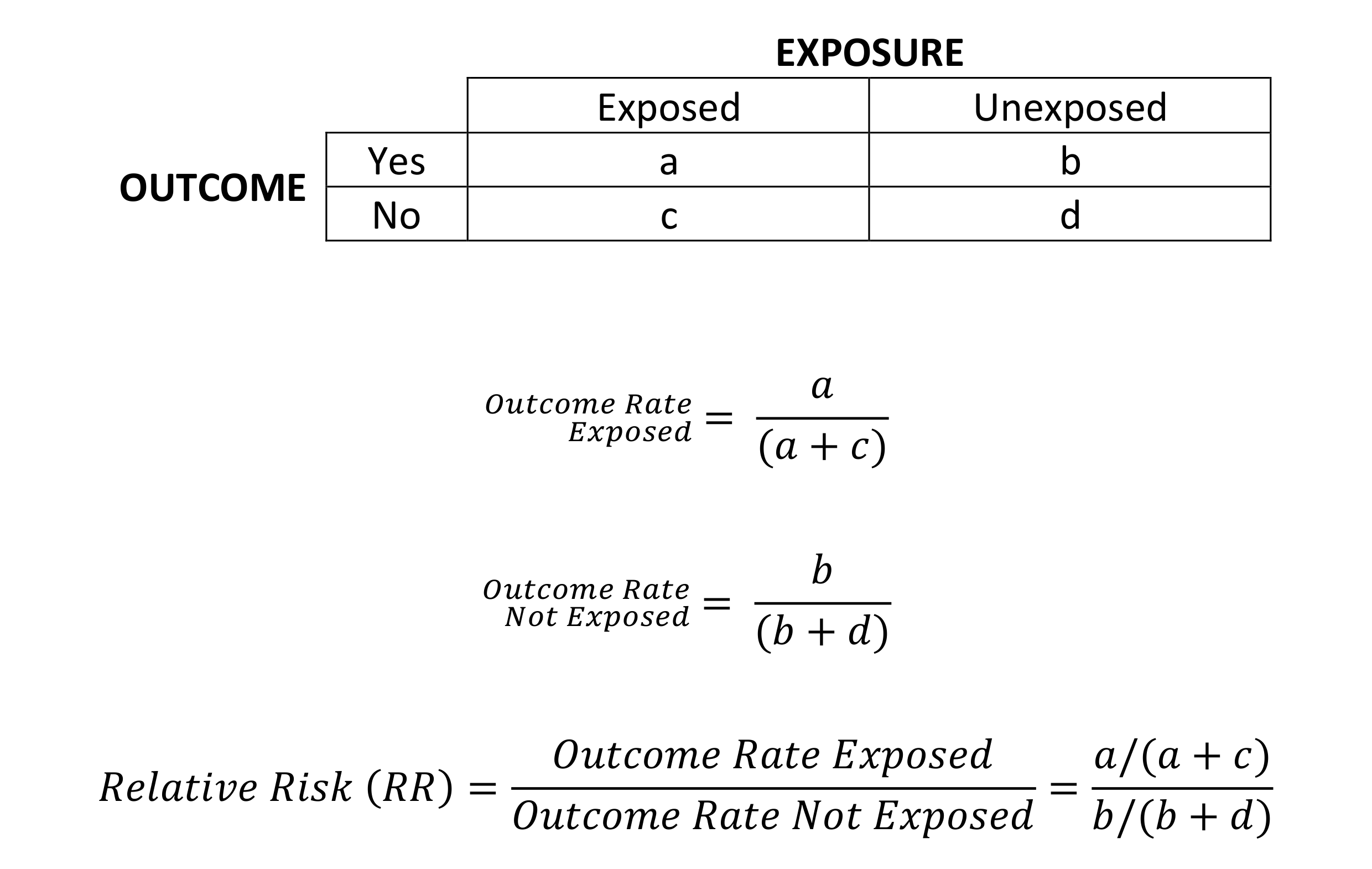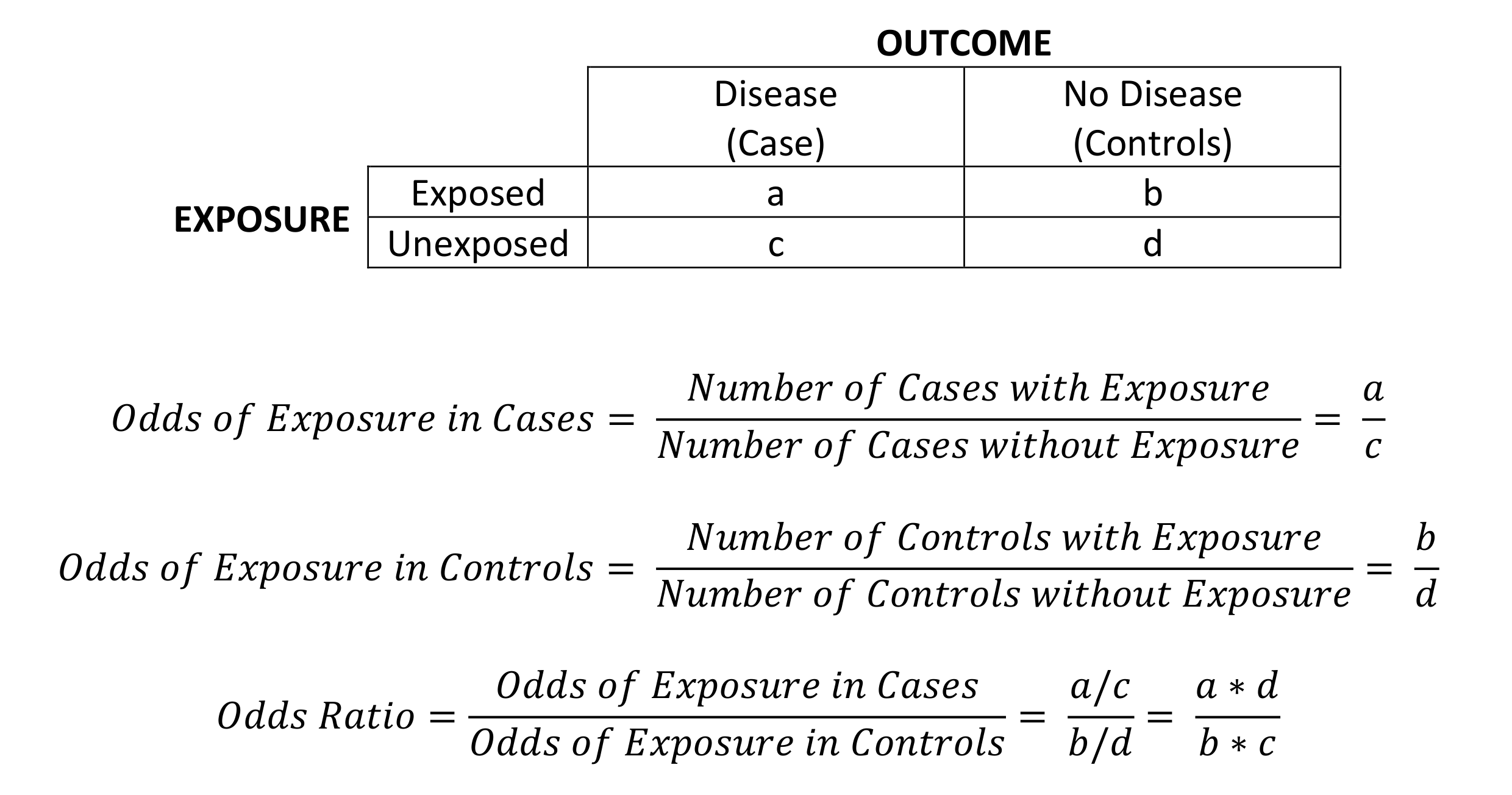# Relative Risk and Odds Ratios

The relative risk and odds ratio are frequently confused for one another, but why? It is probably because both the relative risk and the odds ratio measure association between a binary outcome variable (disease presence or absence) and a binary predictor variable (risk factor presence or absence). Despite these similarities they are calculated differently and therefore must be interpreted differently. In simple terms, the relative risk is a ratio of two probabilities while the odds ratio is the ratio of two odds.

## Relative Risk (Risk Ratio)

The relative risk is a measure of association. It is a ratio of two probabilities, both of the same outcome, but under different conditions. It involves examining the probability of an outcome (such as disease) given the incidence of that outcome in relation to an exposure (suspected risk or protection factor). Essentially, relative risk is a ratio of disease rates among the exposed and unexposed groups. This ratio tells you how much more likely it is for people exposed to a risk/protection factor to develop the disease compared to people who are not exposed.

It is commonly used in prospective studies such as cohort studies. A cohort study involves following groups of people with different characteristics to observe whether or not a particular outcome occurs. You can calculate relative risk as follows:### Interpretation

• If the relative risk is 1, then the risk of disease is similar in both exposed and unexposed groups and the exposure is not associated with the disease.
• If the relative risk is greater than 1, then the exposure is considered to be positively associated with having the disease and could be a risk factor.
• If the relative risk is less than 1, then the exposure is considered to be negatively associated with having the disease and could be a protective factor.

## Odds Ratio

The odds ratio is a measure of association between a particular exposure (suspected risk or protection factor) and a particular outcome (such as disease). It is a ratio of two odds and it tells you how the presence or absence of the exposure has an effect on the presence or absence of the outcome. The odds ratio can then be used to compare the various risk factors for that outcome.

The odds ratio is commonly used in retrospective studies such as case-control studies. This is because the relative risk cannot be calculated and so the odds ratio is used as a point estimate of the relative risk.### Interpretation

• If the odds ratio is 1, then the odds of exposure among cases is the same as the odds of exposure among controls. This means the exposure is not associated with the disease.
• If the odds ratio is greater than 1, then the odds of exposure among cases is greater than the odds of exposure among controls. Therefore, the exposure may be a risk factor for the disease.
• If the odds ratio is less than 1, then the odds of exposure among cases is lower than the odds of exposure among controls. Therefore, the exposure may be a protective factor against the disease.